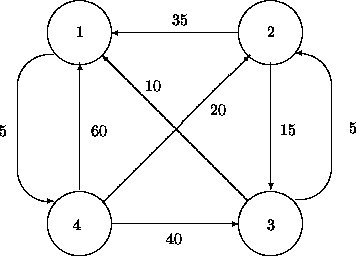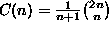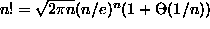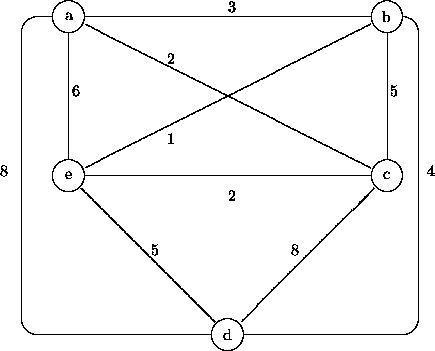## Data Structures and Algorithms 2, Fall 1998 Problem Set 3 (23-25 Nov.)

1. Modify Floyd's algorithm (p. 67 of the lecture notes) so that in addition to computing the shortest distances between nodes the algorithm also makes it possible to actually trace the optimal paths. (Hint: Augment the distance matrix D by another matrix N that keeps track, for each pair of nodes (i,j), which is the node following the start node i on the shortest route leading to j.)
2. Simulate the behaviour of your algorithm using as input the graph below:1. Give a recurrence equation that describes the number C(n) of binary trees with n nodes, and based on this, a reasonably efficient algorithm for computing values of C(n). (Hint: A binary tree consists of a root and a left and a right subtree.)
2. Using the method of ``generating functions'' (e.g. Cormen/Leiserson/Rivest, p. 262) one can actually solve the above recurrence in closed form and obtain the explicit formula. (The numbers C(n) are for historical reasons known as ``Catalan numbers''.) Estimate the order of growth of the function C(n) using Stirling's formula.
1. Try to modify Dijkstra's algorithm (p. 70 of the lecture notes) to find the longest (noncyclic) path connecting two nodes in a graph. Which difficulty do you meet in the algorithm? (Additional information: The longest path problem is NP-complete, and thus probably not solvable by any polynomial-time algorithm.)
1. Simulate Kruskal's algorithm (p. 73 of the lecture notes) for finding a minimal spanning tree of the graph below.2. Simulate the behaviour of the greedy TSP heuristic (p. 75 of the notes) on the same input graph.

Pekka Orponen
Wed Nov 18 02:25:03 EET 1998